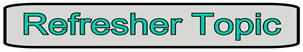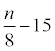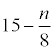Basic Algebraic Expression Practice Terms of Use    Contact Person: Donna RobertsDirections: Choose the best answer as an algebraic expression for each verbal statement. Answer ALL of the questions (or only a few at a time), then use the CHECK ANSWER button at the bottom of the page to see your progress. If you see that an answer is incorrect, go back and redo that question and CHECK ANSWERS again.

1.  Four more than six times a number.
   6 + 4n   4 + 6n   6(4n)   4(6n)

2. The product of twenty and a number.
   20 + n   20 - n   20n   20/n

3. The sum of triple a number and the product of four and five.
   3n (4•5)   3n (4 + 5)   (3 + n) • (4•5)   3n + 4•5

4.  Twelve less than the product of seven and a number.
    7n - 12   12 - 7n   7 + n - 12   12 - (7 + n)

5.  The number of inches in m feet.
    6m    12m   24m   36m

6.  Fifteen less than a number divided by 8.8n - 1515 - 8n

7.  Ian has 67 baseball cards. If he buys c cards each week for w weeks, what is the total number of cards he will have?
   67cw   67 + cw   67c + w   67 + c + w

8.  The perimeter of a square with a side of x + 3.
   x2 + 9   x2 + 6x + 9   4x + 12   4x + 3

9.  Colin has 3 more CDs than Angela. Harley has twice as many CDs as Colin. If n represents the number of CDs owned by Angela, express the CDs owned by Harley.
   2n + 3   2(n + 3)   3(n + 2)   2 + (n + 3)

10.  Two numbers are consecutive even integers. If the smaller number is a + 8, express the larger number.
   a + 10   a + 9   2(a + 8)   a + 6